Continuing with the next best hand after a pair:

 A B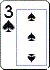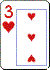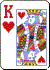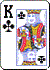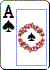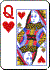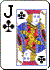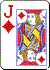Here you have two pair. It is the highest pair which is the deciding factor.

Therefore hand A (Pair of Kings, pair of Threes) beats hand B (pair of Queens, pair of Jacks).

If two hands have the same two pair it is how high the fifth card (or kicker) is which is the deciding factor.

Continuing with the next best hand after two pair:

 A B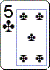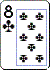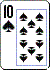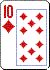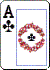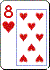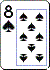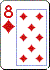Here you have three of a kind (also known as trips or a set). As with a pair, it is the highest three of a kind that wins.

Therefore hand A (three Tens) beats hand B (three Eights).

If two hands have the same three of a kind, it is the kicker which is the deciding factor. This is a possibility in games such as Hold'em, as will be seen later.